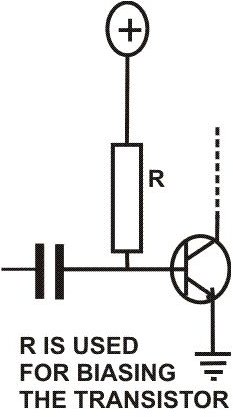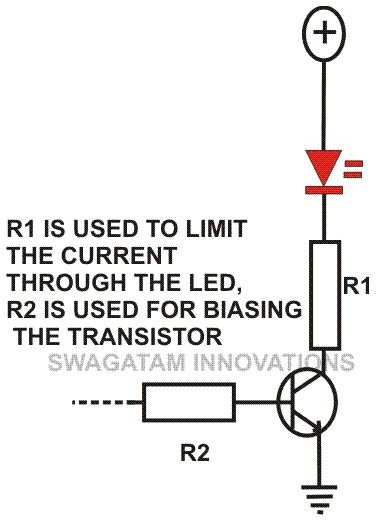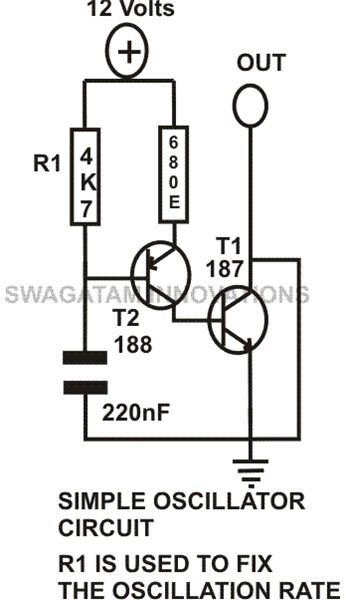# What is the Function of a Resistor? Functions Explained with Illustrations

Page content

## Introduction

Resistors come under passive electronic components and are extensively used in electronic circuits. So important are these components that it may be virtually impossible to build an electronic circuit without involving resistors. Basically the function of a resistor is always to oppose the flow of current through it and the strength of this opposition is termed as its resistance. German physicist, Sir G.S. Ohms was able to discover a definite relationship between voltage, current and resistance. According to him a potential difference or a voltage (V) across a resistor (R) is proportional to the instantaneous current (I) flowing through it and is given as:

V = R.I

Here R is the constant of proportionality and is known as the resistance of the resistor.

## Function of Resistors in Electronics

In electronic circuits, resistors play an important role to limit the current and provide only the required biasing to the vital active parts like the transistors and the ICs. We will try to find out what is the function of a resistor in electronics through the following illustrations:Transistor Biasing: Through one of my previous articles you must have acquired a good knowledge regarding transistors. A transistor basically needs a small base voltage (>0.6) to make a large voltage flow through its collector/ emitter terminals. But the base of a transistor is quite vulnerable to high currents, so a resistor is incorporated here to limit the current and provide a safe biasing voltage.

The value of the base resistor of a transistor may be calculated through the below given formula:

R = (V – 0.6).Hfe / I,

Here V = source voltage to the base resistor, I = the collector load current, Hfe = forward gain of a transistor (150 nominal) and 0.6 = minimum transistor biasing voltage.LED Current Limit: Just like transistors, LEDs too are very sensitive to high currents. A resistor when placed in series with the LEDs regulates a proper flow of current through them. To calculate the value of a series LED resistor, the following formula may be used:

R = V –( N.VLED) / I

Here R = Series LED resistor, V = supply voltage, N = number of LEDs in series, V(LED) = forward voltage of the LED used, and I = current through the LEDs (10mA optimum).In Timing Circuits: The timing components used in timer and oscillator circuits always incorporate a resistor and a capacitor. Here the time taken to charge or discharge a capacitor constitutes the basic time pulse or trigger for the circuit. A resistor is effectively used to control this charging and discharging process and its value is varied to obtain different time intervals.

Surge Protection: The initial switch ON of a power supply may at times inflict a dangerous voltage surge into an electronic circuit, damaging its critical components. A resistor when introduced in series with the supply terminals of the circuit helps in checking the sudden rise in voltage and averting a possible harm. These resistors are generally of low values so that the over all performance of the circuit is not affected.

The above basic examples must have provided you sufficient knowledge regarding the use of resistors in electronic circuits and helped you to understand what is the function of a resistor. For further information, feel free to add your comments (comments need moderation and may take time to appear).